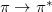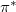Q-Chem 5.0 User’s Manual

# 6.4 Maximum Overlap Method (MOM) for SCF Excited States

The Maximum Overlap Method (MOM)  is a useful alternative to CIS and TDDFT for obtaining low-cost excited states. It works by modifying the orbital selection step in the SCF procedure. By choosing orbitals that most resemble those from the previous cycle, rather than those with the lowest eigenvalues, excited SCF determinants are able to be obtained. The MOM has several advantages over existing low-cost excited state methods. Current implementations of TDDFT usually struggle to accurately model charge-transfer and Rydberg transitions, both of which can be well-modeled using the MOM. The MOM also allows the user to target very high energy states, such as those involving excitation of core electrons , which are hard to capture using other excited state methods.

In order to calculate an excited state using MOM, the user must correctly identify the orbitals involved in the transition. For example, in atransition, theandorbitals must be identified and this usually requires a preliminary calculation. The user then manipulates the orbital occupancies using the $occupied section, removing an electron from theand placing it in the. The MOM is then invoked to preserve this orbital occupancy. The success of the MOM relies on the quality of the initial guess for the calculation. If the virtual orbitals are of poor quality then the calculation may ‘fall down’ to a lower energy state of the same symmetry. Often the virtual orbitals of the corresponding cation are more appropriate for using as initial guess orbitals for the excited state. Because the MOM states are single determinants, all of Q-Chem’s existing single determinant properties and derivatives are available. This allows, for example, analytic harmonic frequencies to be computed on excited states. The orbitals from a Hartree-Fock MOM calculation can also be used in an MP2 calculation. For all excited state calculations, it is important to add diffuse functions to the basis set. This is particularly true if Rydberg transitions are being sought. For DFT based methods, it is also advisable to increase the size of the quadrature grid so that the more diffuse densities are accurately integrated. Example 6.117 Calculation of the lowest singlet state of CO. $comment
CO spin-purified calculation
$end$molecule
0 1
C
O  C  1.05
$end$rem
METHOD            B3LYP
BASIS             6-31G*
$end @@@$molecule
$end$rem
METHOD            B3LYP
BASIS             6-31G*
MOM_START         1
UNRESTRICTED      true
OPSING            true
$end$occupied
1 2 3 4 5 6 7
1 2 3 4 5 6 8
$end  The following$rem is used to invoke the MOM:

MOM_START
 Determines when MOM is switched on to preserve orbital occupancies.

TYPE:
 INTEGER

DEFAULT:
 0 (FALSE)

OPTIONS:
 0 (FALSE) MOM is not usedMOM begins on cycle.

RECOMMENDATION:
 For calculations on excited states, an initial calculation without MOM is usually required to get satisfactory starting orbitals. These orbitals should be read in using SCF_GUESS = true and MOM_START = 1.

Example 6.118  Input for obtaining theAexcited state of formamide corresponding to thetransition. TheAground state is obtained if MOM is not used in the second calculation. Note the use of diffuse functions and a larger quadrature grid to accurately model the larger excited state.

$molecule 1 2 C H 1 1.091480 O 1 1.214713 2 123.10 N 1 1.359042 2 111.98 3 -180.00 H 4 0.996369 1 121.06 2 -0.00 H 4 0.998965 1 119.25 2 -180.00$end

$rem METHOD B3LYP BASIS 6-311(2+,2+)G(d,p) XC_GRID 000100000194$end

@@@

$molecule 0 1 C H 1 1.091480 O 1 1.214713 2 123.10 N 1 1.359042 2 111.98 3 -180.00 H 4 0.996369 1 121.06 2 -0.00 H 4 0.998965 1 119.25 2 -180.00$end

$rem METHOD B3LYP BASIS 6-311(2+,2+)G(d,p) XC_GRID 000100000194 MOM_START 1 SCF_GUESS read UNRESTRICTED true$end

$occupied 1:12 1:11 13$end


Additionally, it is possible to perform a CIS/TDDFT calculation on top of the MOM excitation. This capability can be useful when modeling pump-probe spectra. In order to run MOM followed by CIS/TDDFT, the $rem variable cis_n_roots must be specified. Truncated subspaces may also be used, see Section 6.3.2. Example 6.119 MOM valence excitation followed by core-state TDDFT using a restricted subspace $molecule
0 1
O    0.0000    0.0000    0.1168
H    0.0000    0.7629   -0.4672
H    0.0000   -0.7629   -0.4672
$end$rem
METHOD       B3LYP
BASIS        aug-cc-pvdz
SYMMETRY     false
SYM_IGNORE   true
$end @@@$molecule
$end$rem
METHOD         B3LYP
BASIS          aug-cc-pvdz
MOM_START      1
UNRESTRICTED   true
SYMMETRY       false
SYM_IGNORE     true
CIS_N_ROOTS    5
TRNSS          true  ! use truncated subspace for TDDFT
TRTYPE         3     ! specify occupied orbitals
CUTVIR         15    ! truncate high energy virtual orbitals
N_SOL          1     ! number core orbitals, specified in $solute section$end

$solute 1$end

$occupied 1 2 3 4 5 1 2 3 4 6$end


If the MOM excitation corresponds to a core hole, a reduced subspace must be used to avoid de-excitations to the core hole. The $rem variable CORE_IONIZE allows only the hole to be specified so that not all occupied orbitals need to be entered in the$solute section.

CORE_IONIZE
 Indicates how orbitals are specified for reduced excitation spaces.

TYPE:
 INTEGER

DEFAULT:
 1

OPTIONS:
 1 all valence orbitals are listed in $solute section 2 only hole(s) are specified all other occupations same as ground state RECOMMENDATION:  For MOM + TDDFT this specifies the input form of the$solute section. If set to 1 all occupied orbitals must be specified, 2 only the empty orbitals to ignore must be specified.

Example 6.120  O(1s) core excited state using MOM followed by excitations among valence orbitals. Note that a reduced excitation subspace must be used to avoid “excitations” into the empty core hole

$molecule 0 1 O 0.0000 0.0000 0.1168 H 0.0000 0.7629 -0.4672 H 0.0000 -0.7629 -0.4672$end

$rem METHOD B3LYP BASIS aug-cc-pvdz SYMMETRY false SYM_IGNORE true$end

@@@

$molecule read$end

$rem METHOD B3LYP BASIS aug-cc-pvdz SCF_GUESS read MOM_START 1 UNRESTRICTED true SYMMETRY false SYMMETRY_IGNORE true CIS_N_ROOTS 5 TRNSS true ! use truncated subspace for TDDFT TRTYPE 3 ! specify occupied orbitals N_SOL 1 ! number core holes, specified in$solute section
CORE_IONIZE       2     ! hole orbital specified
$end$solute
6
$end$occupied
1 2 3 4 5
2 3 4 5 6
\$end